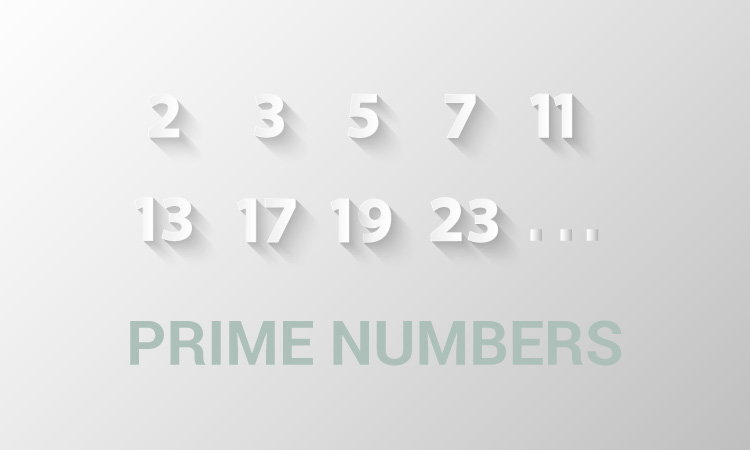C Program to Check Whether a Number can be Expressed as Sum of Two Prime Numbers

Example to check if an integer (entered by the user) can be expressed as the sum of two prime numbers of all possible combinations with the use of functions.To understand this example, you should have the knowledge of following C programming topics:

To accomplish this task, checkPrime() function is created.

The checkPrime() returns 1 if the number passed to the function is a prime number

Example: Integer as a Sum of Two Prime Numbers

#include <stdio.h>
int checkPrime(int n);
int main()
{
int n, i, flag = 0;

printf("Enter a positive integer: ");
scanf("%d", &n);

for(i = 2; i <= n/2; ++i)
{
// condition for i to be a prime number
if (checkPrime(i) == 1)
{
// condition for n-i to be a prime number
if (checkPrime(n-i) == 1)
{
printf("%d = %d + %d\n", n, i, n - i);
flag = 1;
}

}
}

if (flag == 0)
printf("%d cannot be expressed as the sum of two prime numbers.", n);

return 0;
}

// Function to check prime number
int checkPrime(int n)
{
int i, isPrime = 1;

for(i = 2; i <= n/2; ++i)
{
if(n % i == 0)
{
isPrime = 0;
break;
}
}

return isPrime;
}

Output

Enter a positive integer: 34
34 = 3 + 31
34 = 5 + 29
34 = 11 + 23
34 = 17 + 17